# Basic and Complex Uses of Not Equal Comparison Operator in T-SQL

Total: 9 Average: 3.6

The article also highlights the importance of understanding the correct use of Not Equal comparative operator with expressions.

The Not Equal comparative operator is a very common operator used in T-SQL, however, it is important to understand how to use it effectively in different scenarios.

## Basics of Not Equal <> Comparison Operator

Let us first get familiar with the Not Equal T-SQL operator in the light of Microsoft documentation.

### Microsoft Definition

A Not Equal To <> is a comparison operator which compares two expressions and returns a Boolean type result.

### Boolean Type Result

Boolean type result is the type of result which returns True or False.

In the context of T-SQL under normal circumstances, Boolean type returns one of the three values:

1. True
2. False
3. NULL

### Comparison Operator

A comparison operator in simple words compares two expressions and based on the comparison it tells us whether they are same or not.

Please note that Not Equal comparison operator (<>) is a binary operator which means an operator which compares two expressions.

### Expression

According to Microsoft documentation, an expression is a combination of symbols and operators that the SQL Server Database Engine evaluates to obtain a single data value.

Simple expressions can be a single constant, variable, column, or scalar function.

In other words, the simplest form of expression is a constant (fixed number) such as 1.

### Example –Operator and Expressions

The simplest example of an expression with operators is an arithmetic operation such as 1+2.

Would you like to guess what is the operator and what is the expression in the above example?

Please refer to Not Equal comparison operator (<>) definition which states, “Not Equal comparison operator (<>) compares two expressions…” so + (addition) is the operator in this example and 1 (constant) and 2 (constant) are expressions.

### Example – Comparison Operator and Expressions

Considering the example of arithmetic operation 1+2, simply replacing the arithmetic operator + with Not Equal <> comparison operator, it becomes a comparison operation in T-SQL.

For example, 1<>2 is a comparison operation with comparison operator <> between two expressions 1 and 2.

### Not Equal Operator “<>” vs “!=”

There is another way to express Not Equal comparison operator and that is by using exclamation mark followed by equal sign (!=).

Please note that both “<>” and “!=” represent Not Equal comparison operator, except that the <> sign complies with the ISO standard, whereas the Not Equal comparison operator != does not comply with the ISO standard.

### Compatibility

According to Microsoft documentation, the Not Equal comparison operator (<>) is compatible with the following SQL database versions:

1. SQL Server 2008 and upper versions
2. Azure SQL Database
3. Azure SQL Data Warehouse
4. Parallel Data Warehouse

### Syntax

expression (constant, variable, column etc.) <> expression (constant, variable, column etc.)

## Pre-requisites

### T-SQL Familiarity

This article assumes that the readers have basic knowledge of  T-SQL and are capable of writing and running basic SQL scripts.

### Setup Sample Database (SQLDevBlogV2)

This article requires the following sample database to run examples of basic and advanced uses of the Not Equal comparison operator (<>).

The sample database consists of three tables:

1. Article
2. Author
3. Category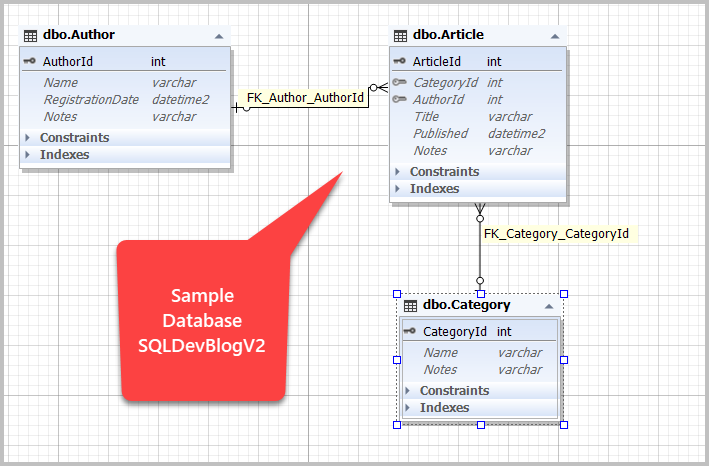Please set up the sample database named SQLDevBlogV2 by writing the following code:

## Basic Uses of Not Equal Operator (<>)

In this section, we are going to explore some basic uses of the Not Equal comparison operator (<>) in T-SQL.

Please remember that the upcoming examples run against the sample database SQLDevBlogV2 which must be created if you would like to run the scripts of examples as mentioned in the pre-requisites.

### Authors list excluding the first author

We have the Author table in the sample database which contains a list of all the registered authors.

Let us first view all the authors as follows: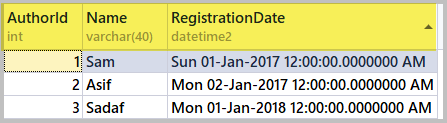Now, if we want to view all the authors except the first one, we are going to use the Not Equal comparison operator (<>) as follows: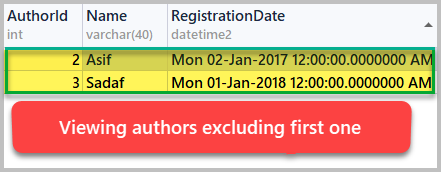Here, the Not Equal comparison operator (<>) is used to filter the results based on the desired criteria.

Please note that in the WHERE clause of the SQL script (where AuthorId<>1), the AuthorId being a column that fulfills the definition of the expression and 1 is a constant, is also an expression so the use complements its syntax (expression <> expression).

### Authors list not registered in 2017

Let us say we want to see the list of authors who were not registered in 2017.

This can be achieved by using the Not Equal comparison operator (<>) as follows: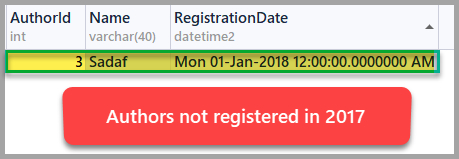Keeping the expression <> expression syntax in mind, this time expression on the left Year(RegistrationDate) is a function that returns year (number) compared with the expression on the right which is 2017 (number).

Please remember that we have already mentioned that the simplest form of expression is a number and in this example, we saw it in action.

### Articles list excluding testing category

If you are interested to see the list of all the articles except for the testing category stored in the sample database then you would require the Not Equal comparison operator (<>).

If you refer to the sample database diagram at the beginning of this article, it is obvious that the Article table is connected with Category through foreign key relationship, so, first of all, we need to see the categories with articles by using INNER JOIN as follows: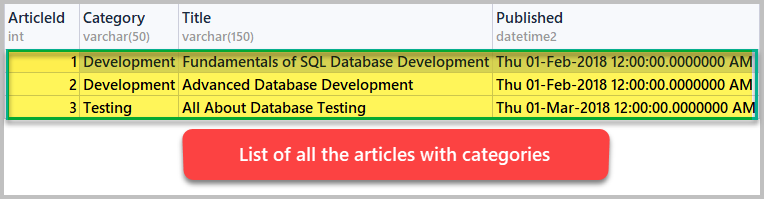Now, we need to exclude the Testing category to see all the articles except the testing articles using the Not Equal comparison operator (<>) as follows:

Running the script produces the following output: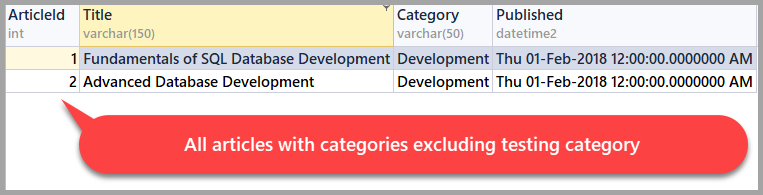## Complex Uses of Not Equal comparison operator (<>)

We are now going to look at some slightly complex examples, which involve the use of the Not Equal comparison operator (<>).

### Authors list excluding current year authors

A slightly complex scenario is when you are asked to get all the authors except those who were registered in the current year.

Since the current year is dynamic and it changes from year to year, this makes the script slightly complex as we cannot simply use a fixed year number with the comparison operator.

The solution is to get the current year from the current date and then get the list of all the authors who were not registered in the current year.

This is achieved by the following code:

The result is as follows: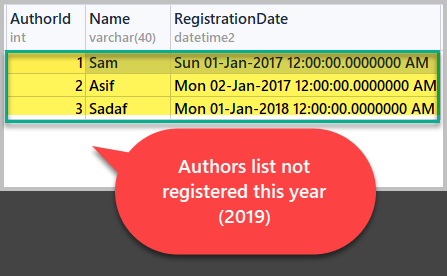Please note that since all the authors in the sample database were registered in 2017 and 2018 so all the authors are shown without any exclusion.

### Authors list excluding the author(s) with most articles

The requirement is to view the authors’ list without the top author(s) makes the script slightly more complex.

We have to divide the problem into smaller parts:

1. Getting top author(s) (author with most articles)
2. Excluding top author(s) using the Not Equal comparison operator (<>)
3. Viewing all authors excluding top author(s)

The top author is an author who has more written articles than any other author.

Let us first add one more article for the author Sadaf to make her the top author by running the following script:

Now, build a script to view authors with the total number of articles ordered by authors with most articles first:

The output of the script is as follows: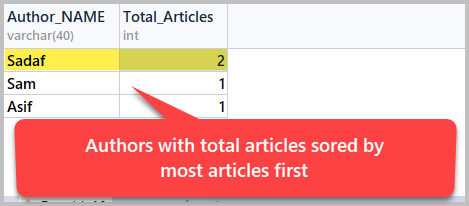Now first we need to find out the most articles by an author and put the result into a variable and then we can list all the authors excluding the ones with most articles by using Not Equal comparison operator (<>) with the variable as follows:

Running the script shows us the following result set: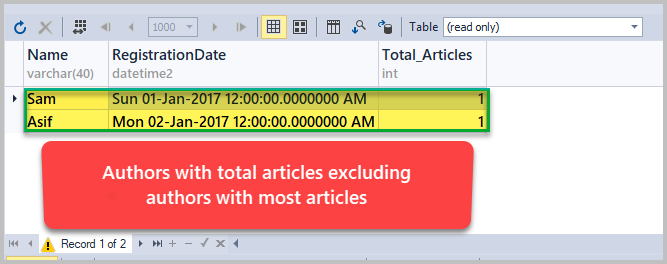Congratulations, you have successfully learned how to use the Not Equal comparison operator (<>) in simple and slightly complex scenarios.

## Things to do

Now that you are familiar with basic and complex uses of the Not Equal comparison operator (<>), you can improve your skills further by trying the following things:

1. Please try to find out the list of all the articles excluding the articles which were written last year, which should be calculated dynamically.
2. Please try to create SQL script to get the list of all the authors who were not registered last year, where the last year should be calculated dynamically.
3. Please try finding out the list of all the articles, where the category was not development using the sample database created in this article.
4. Please try to build a SQL script to get all the categories excluding the top category (category with most articles written).
5. Please try creating a scalar-valued function and use it with the Not Equal comparison operator (<>) to explore further.

### Haroon Ashraf

Haroon's deep interest in logic and reasoning at an early age of his academic career paved his path to become a data professional.

He began his professional life as a computer programmer more than 10 years ago working on his first data venture to migrate and rewrite a public sector database driven examination system from IBM AS400 (DB2) to SQL Server 2000 using VB 6.0 and Classic ASP along with developing reports and archiving many years of data.

His work and interest revolves around Database-Centric Architectures and his expertise include database and reports design, development, testing, implementation and migration along with Database Life Cycle Management (DLM).

#### Latest posts by Haroon Ashraf (see all)